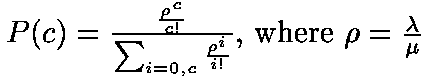# A Robust and Efficient Algorithm for Evaluating Erlang's Formula -- Erlang B --

June 1998

Note: This is a companion page to Qiao and Qiao paper, 1998. (download ps, pdf)

### Introduction

In the 1920's, a Danish mathematician and telephone traffic engineer named A. K. Erlang studied delays in telephone traffic and came up with several models which are still in use today. These models deal with the following terms:

Arrival Rate - lambda
The mean number of arrivals per unit time. If five hundred calls arrive in an hour, on average, then the Arrival Rate is 500 calls/hour. The reciprocal (1/lambda) is then the average amount of time that seperates incoming calls.
Service Rate - mu
The mean number of calls serviced per unit time. If it takes 20 minutes to service one call, then 3 calls may be serviced in one hour and the Service Rate is 3 calls/hour. The reciprocal (1/mu) is the average time taken to service one call, given in units of time per call (ie. 0.333 hours/call or 20 minutes/call in the current example)
Number of Lines - c
The number of independent phone lines. This determines the number of calls that can be serviced concurrently.
Capacity
The size of the call queue. This represents the total number of calls that can be serviced at a given time as well as the total number of calls that can be held until a line becomes free. Notice that in a system with no holding queue, the capacity is equal to the number of lines.
Busy Hour
The busiest, one-hour period of the day. This is the period when incoming calls are most likely to be blocked or turned away, so this is the time for which statistics are calculated.
Busy Hour Traffic (BHT) - rho
The amount of traffic in the system during the Busy Hour. This can be measured in several ways, including call minutes and call hours. In our example system, where 500 calls come in per hour and each call takes 20 minutes (0.333 hours) to service, then the system has to handle 500 * 0.333 = 166.5 call hours during the busiest hour of the day.
Erlang Rho>
Another common unit is the Erlang, representing the ratio of Arrival Rate to Service Rate (lambda/mu). For example if we expect 500 calls/hour, the Arrival Rate lambda = 500. If the average duration of a call is 20 minutes, or 0.333 hours, then the Service Rate mu = 3. BHT is then calculated as lambda/mu = 500/3 = 166.67
The percentage of incoming calls turned away during the Busy Hour because all lines are busy at the time of the call. Notice that this statistic is a function of the number of lines, with more lines resulting in a lower and therfore better Grade of Service.

### Erlang B

The Erlang B model deals with a traffic system in which:

• Arrival Rate is Poisson distribution with parameter lambda (l);
• Service Rate is exponential with parameter mu (u);
• Number of Lines is c;
• Capacity is also c.
This model allows one to easily calculate the Grade of Service by the following formula:Other common usages include calculating various traffic load statistics such as the maximum service time and the minimum number of phone lines necessary to maintain a given Grade of Service.

Traditional, straightforward evaluation of the given equation results in overflow errors unless rho and c are relatively small (between 100 and 200). To circumvent these problems, we can reformulate the Erlang B formula as:Implementing this rewritten formula efficiently vastly reduces the magnitude of computation, thus allowing larger values for the system. A more detailed discussion of this approach can be found in the companion paper, Qiao and Qiao (1998).

### The Calculator

This calculator will handle systems at least as large as rho=1e8,c=1e7, but become prohibitively slow as the systems's magnitude increases. The calculation time becomes noticable when (rho*c) exceeds 10^7. If the calculator's behaviour becomes confusing, clear the fields and try again.

#### The Calculator with commonly used input and output fields

 Arrival Rate - l (# arrivals / unit time) Service Rate - u (# services / unit time) GradeOfService (failure rate) Result: Capacity/Num of Lines ...

#### The Calculate with more features

• Calculate button will calculate the specified value for the corresponding inputs.
• Clear All Values button will clear all values in every fields.
• Use Exist Values button will allow you to use already inputed or calculated values for other input fields.
 Arrival Rate - l (# arrivals / unit time) Service Rate - u (# services / unit time) Result: Erlangs - rho

 Erlangs - rho Grade of Service - P(c) Result: Capacity/Num of Lines
 Arrival Rate - l (# arrivals / unit time) Service Rate - u (# services / unit time) Grade Of Service - P(c) Result: Capacity/ Num of Lines

 Erlangs - rho Capacity/ Num of Lines Result: Grade of Service

### References

All algorithms on this page came from Qiao and Qiao (1998) while the Intel Support page was used solely for definitions.

Page originally written by Dave Albert, modified by Jun Zhu
Last updated on Mar. 13 2000